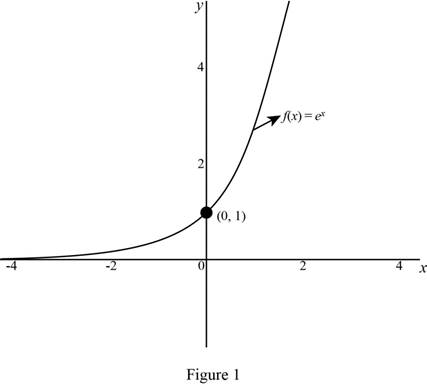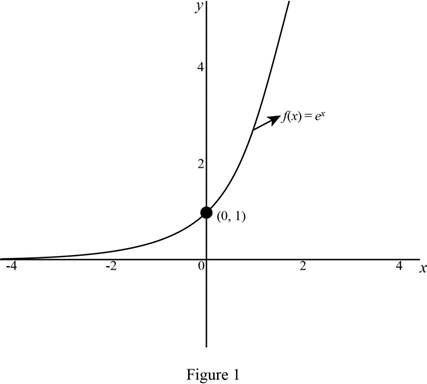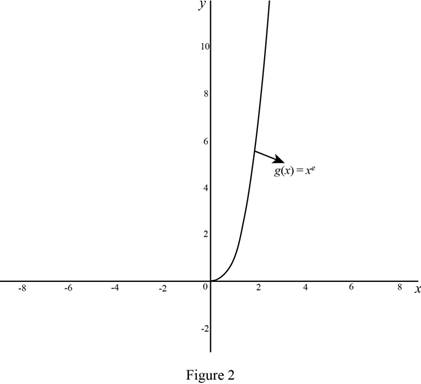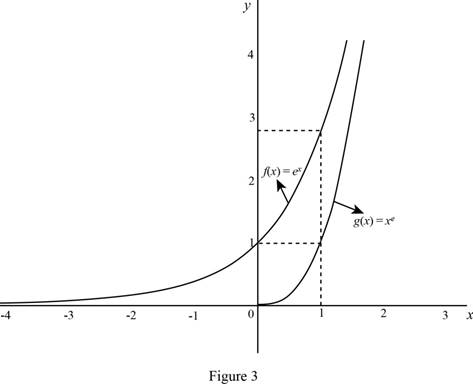# The graph of the function and obtain the point when the function crosses the y -axis.### Single Variable Calculus: Concepts...

4th Edition
James Stewart
Publisher: Cengage Learning
ISBN: 9781337687805### Single Variable Calculus: Concepts...

4th Edition
James Stewart
Publisher: Cengage Learning
ISBN: 9781337687805

#### Solutions

Chapter 3.1, Problem 2E

(a)

To determine

## To sketch: The graph of the function and obtain the point when the function crosses the y-axis.

Expert Solution

The graph of the function f(x)=ex is shown below in Figure 1.The function f(x)=ex crosses the y axis at (0,1).

### Explanation of Solution

Given:

The function is f(x)=ex.

Formula used: Derivative of Exponential Function

ddx(ex)=ex (1)

Calculation:

Obtain the derivative of f(x)=ex.

Use the derivative of exponential function in equation (1).

f(x)=ex

Since the given function and its derivative are same, f(x)=f(x).

The graph of the function f(x)=ex is shown below in Figure 1.From the graph, it is observed that the function f(x)=ex increases for all x, because the derivative of the function f(x)=ex is positive for all x.

Moreover, the function is closer to zero as x approaches minus infinity and it is closer to infinity as x approaches plus infinity.

That is, limxex=0 and limxex=.

Substitute 0 for x in f(x)=ex,

f(0)=e0=1

Therefore, the function is crosses the y axis at (0,1).

(b)

To determine

### To describe: The type of functions f(x) and g(x) and compare the differentiation formulas for f(x) and g(x).

Expert Solution

Both the functions f(x) and g(x) are increasing function, and f(x) is an exponential function.

The differentiation formulas for f(x) and g(x) are f(x)=ex and g(x)=exe1.

### Explanation of Solution

Given:

The function are f(x)=ex and g(x)=xe.

Formula used: Power Rule

If n is a real number, then ddx(xn)=nxn1 (2)

Calculation:

The graph of the function g(x)=xe is shown below in Figure 2.From the graph, it is observed that the function g(x)=xe is defined for only positive value of x and g(x)=exe1 is positive for all x.

Thus, g(x)=xe is an increasing function for all value of x.

From part (a), f(x)=ex is an exponential function which is defined for all value of x and it is an increasing function.

The derivatives of both the functions f(x)=ex and g(x)=exe1 are increasing when x increases.

Therefore, both the functions f(x) and g(x) are increasing functions and f(x) is an exponential function.

Obtain the derivatives of f(x) and g(x).

From part (a), the derivative of f(x) is f(x)=ex.

Since the derivative of g(x) is g(x), g(x)=ddx(xe).

Apply the Power rule (2), g(x)=exe1.

Thus, the derivative of g(x) is g(x)=exe1.

Therefore, the differentiation formulas for f(x) and g(x) are f(x)=ex and g(x)=exe1.

(c)

To determine

### To identify: The function which grows more rapidly when x is large.

Expert Solution

The function f(x)=ex is faster rate compared to g(x)=xe when x is large.

### Explanation of Solution

Given:

The function are f(x)=ex and g(x)=xe.

The graph of the functions f(x)=ex and g(x)=xe is shown below in Figure 3.From the graph, it is observed that the value of f(x)=ex is larger than g(x)=xe when x=1.

That is, f(1)>g(1).

Therefore, the function f(x)=ex grows more rapidly than g(x)=xe when x is large.

### Have a homework question?

Subscribe to bartleby learn! Ask subject matter experts 30 homework questions each month. Plus, you’ll have access to millions of step-by-step textbook answers!